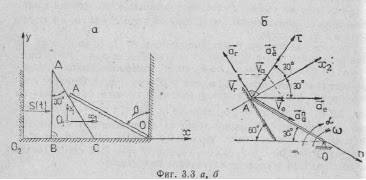# Simple Mechanics, relative motion example

• twowheelsbg
In summary, the problem involves a prism sliding horizontally and a bar rotating with point A sliding over the prism's slope. The task is to find the angular velocity and acceleration of the bar at t=1s, while the bar is declined at β=60deg from the vertical. The equations used are Va=ωxOA and Aa=αxOA+ωxVa, and the solution involves finding Ve and Ae from the prism's horizontal motion, Vr and Ar from the relative motion over the prism's slope, and Va and Aa from the circular path with radius OA. The final results for ω and α are 1.3s-1 and 0.176s-2 respectively, which differ from

## Homework Statement

Might be the sketch most useful first:The prism is sliding horizontally from left to right under condition : S(t)=4*t*t+5*t+1[cm]
and the bar OA is rotating, point A is sliding over the prism's slope and point O is the immobilized center of bar's rotation, OA=10cm.

What would be the angular velocity and acceleration of the bar at the moment t=1s,
if at that moment the bar is declined at β=60deg from the vertical?

## Homework Equations

Va=ωxOA
Aa=αxOA+ωxVa

Idea is the point A is once transferred by the prism's horizontal motion to the left ( Ve and Ae ),
and twice transferred over the prism's slope in relative motion ( Vr and Ar ).
It's absolute motion ( Va and Aa ) comes also from the circular path with radius OA.

Va=Ve+Vr
Aa=Ae+Ar

## The Attempt at a Solution

Ve(t)=dS(t)/dt, so Ve(1s)=13cm/s
point A is following a circle so Va is 60deg above Ox ( τ direction ),
otherwise Vr is parallel to the prism's slope ( η direction ).
This way vector solving of Va=Ve+Vr results in Va=13cm/s, Vr=13cm/s.
Va is perpendicular to OA so ω=Va/OA=1.3s-1
( this is my first problem, examples booklet answer is 1.0s-1 )

Ae(t)=dVe(t)/dt, so Ae(1s)=8cm/s.s ,
Ar is parallel to the prism's slope ( η direction ),
Aa has accelerating part αxOA ( τ direction ) and centripetal part ωxωxOA ( η direction ).
Solving Aa=Ae+Ar in vectors results in α=0.176s-2
( this is my second problem, examples booklet answer is 0.19s-1 )

I could attach my Mathcad sheet if needed.
(My results for Aa=17cm/s.s , Ar=11.5cm/s.s )

Last edited:
twowheelsbg said:

## Homework Statement

Idea is the point A is once transferred by the prism's horizontal motion to the RIGHT ( Ve and Ae ),
and twice transferred over the prism's slope in relative motion ( Vr and Ar ).
It's absolute motion ( Va and Aa ) comes also from the circular path with radius OA.

Later edit, sorry for the typinng bug.